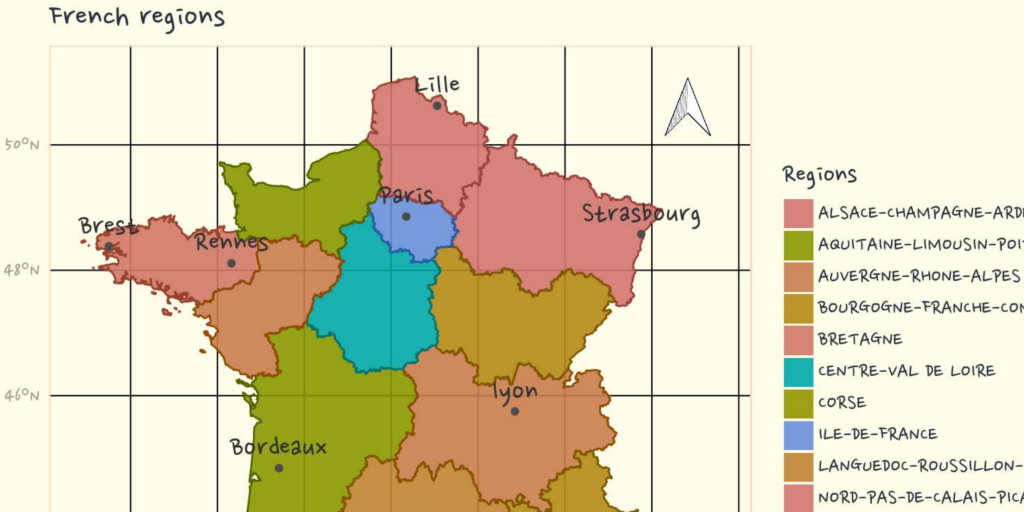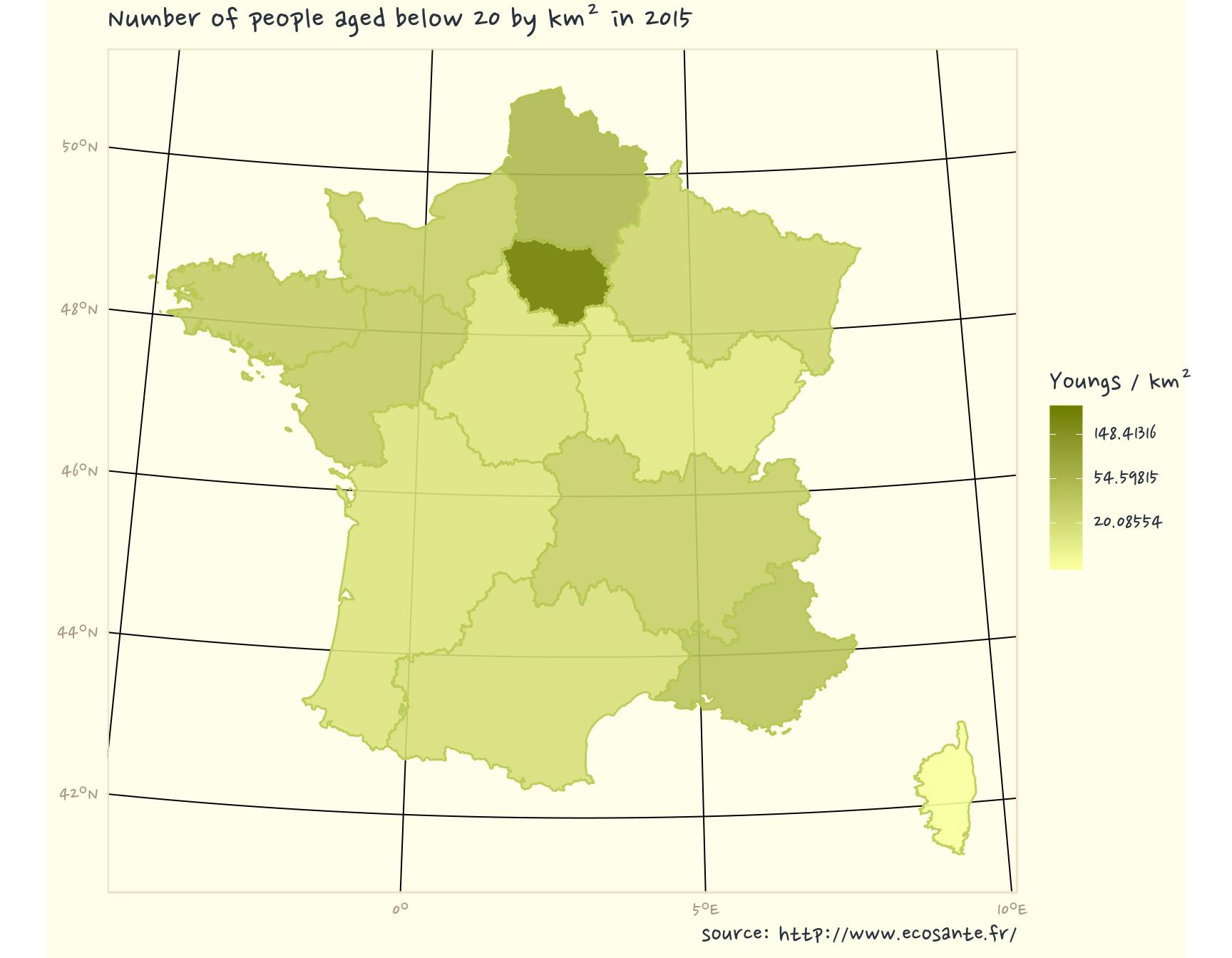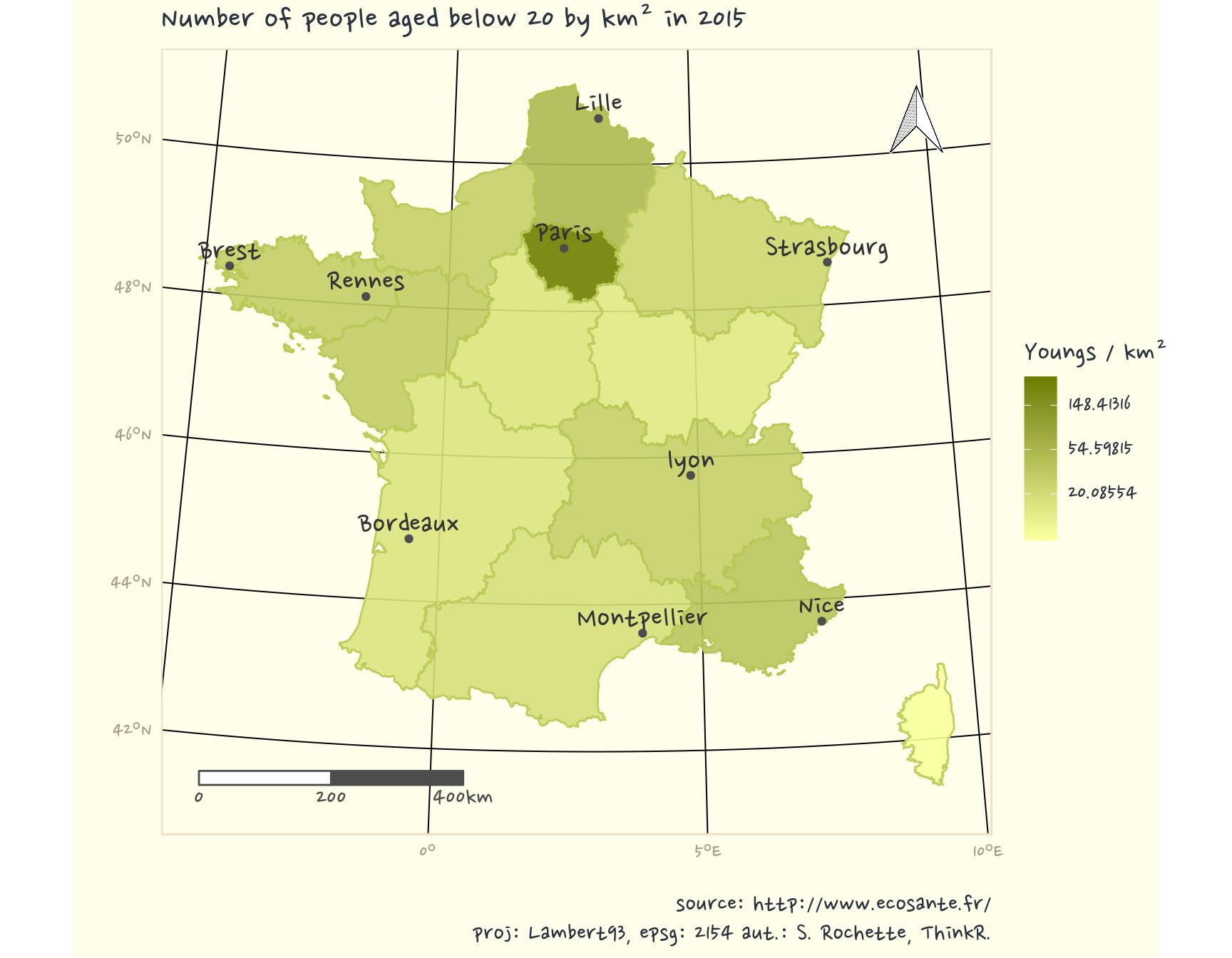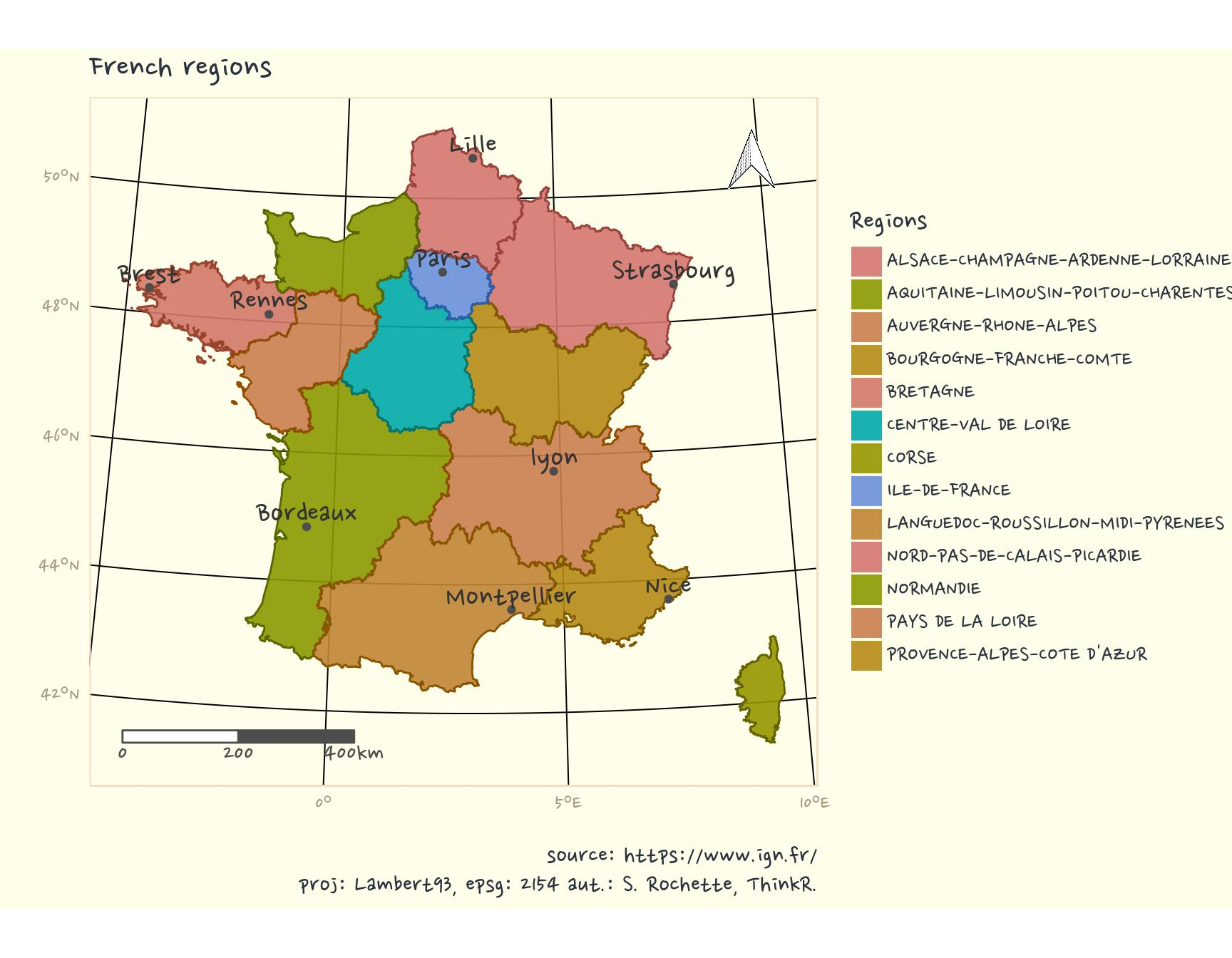# Painting maps: context

On the Grrr Slack, a mutual-aid Slack on R for French speaking people, Thomas Vroylandt showed the following maps asking how this could be done using {ggplot2} :EDIT : This maps was originally presented on visionscarto.net with drawings by Agnès Stienne

I found these maps really nice. As I like drawing maps, indeed, I was drawing French regional maps to prepare a tutorial, I decided to take a few minutes to install the library and change the look of my maps.

## Libraries needed

As of today (2018-04-18):

• You need the development version of {ggplot2} to draw maps using `geom_sf`.
• Package {ggpomological} is on github only.
• You also need my version of {ggsn} (“font-family” branch) if you want a custom font family in `scalebar`. EDIT: Package {ggsn} has been updated

For a full authentic pomological look, you need to install {magick} and a handwriting font. Garrick Aden-Buie proposes a few fonts from Google Fonts:

Personaly, I also like:

I let you find out how to install a new font on your OS and how to make it available in R. You may need `extrafont::font_import()`.

``````# devtools::install_github("tidyverse/ggplot2")
library(ggplot2)
library(ggpomological)
library(scales)
library(sf)
library(dplyr)
library(grid)
# devtools::install_github("statnmap/ggsn", ref="font-family")
library(ggsn)
library(units)
library(extrafont)
# extrafont::font_import()``````

## Painting a first map

Let’s get some data. A map, of course ! I am currently working on a map of French regions with the repartition of the population by age classes.

``````# extraWD <- "."
if (!file.exists(file.path(extraWD, "Region_L93.rds"))) {
githubURL <- "https://github.com/statnmap/blog_tips/raw/master/2018-04-18-draw-maps-like-paintings/Region_L93.rds"
}

We represent the number of people below 20 years old in 2015 by region.

``````ggplot() +
geom_sf(data = Region_L93,
aes(fill = Age_0.19.ans), alpha = 0.75, colour = NA
) +
geom_sf(data = Region_L93,
aes(colour = Age_0.19.ans), alpha = 1, fill = NA
) +
"Youngs (< 20y)",
low = muted(ggpomological:::pomological_palette, l = 90, c = 70),
high = ggpomological:::pomological_palette
) +
low = muted(ggpomological:::pomological_palette, l = 90, c = 70),
high = ggpomological:::pomological_palette,
guide = FALSE
) +## Change font family in {ggplot2} theme

If you downloaded “NanumPenScript” font, we can go further. We change values represented to number per km², which as more sense for comparison. We modify a little the color palette, add title and source.

``````Region_Prop_L93 <- Region_L93 %>%
mutate(area_km2 = st_area(.) %>% set_units(km^2) %>% drop_units()) %>%
mutate(Prop_20_km2 = `Age_0.19.ans` / area_km2)

ggplot() +
geom_sf(data = Region_Prop_L93,
aes(fill = Prop_20_km2), alpha = 0.9, colour = NA
) +
geom_sf(data = Region_Prop_L93,
aes(colour = Prop_20_km2), alpha = 1, fill = NA
) +
"Youngs / km²",
low = muted(ggpomological:::pomological_palette, l = 100, c = 70),
high = muted(ggpomological:::pomological_palette, l = 50, c = 70),
trans = "log"
) +
low = muted(ggpomological:::pomological_palette, l = 80, c = 70),
high = muted(ggpomological:::pomological_palette, l = 50, c = 70),
guide = FALSE
) +
theme_pomological_fancy(base_family = "Nanum Pen") +
labs(
title = "Number of people aged below 20 by km² in 2015",
caption = "source: http://www.ecosante.fr/"
)``````## Add all necessary information on the map

Let’s add some cities coordinates, transform as spatial feature, define coordinate system.
Add north and scalebar. I don’t know when we stopped adding North and scalebar on maps, but I learnt to never forget it. {ggsn} helps us doing it with {ggplot2}. We should also add information on projection.
Good to know : To add some `geom_text` over a map with {sf} data, it may be necessary that coordinates of the text are in the same coordinates system as the projection of the figure. Hence, we will modify “Regions” layer to be in non-projected geographic coordinates (EPSG: 4326).

``````# Add cities and project in Lambert93
cities_L93 <- tibble(
city = c("Paris", "Rennes", "Lille", "Strasbourg", "Brest", "Bordeaux",
"Montpellier", "Nice", "lyon"),
lat = c(48.857256, 48.110867, 50.625291, 48.576816, 48.384679, 44.843019,
43.609519, 43.694233, 45.749206),
long = c(2.344655, -1.678327, 3.057288, 7.754883, -4.498229, -0.581680,
3.877594, 7.245262, 4.847652)
) %>%
st_as_sf(coords = c("long", "lat")) %>%
st_set_crs(4326) %>%
st_transform(2154) %>%
bind_cols(st_coordinates(.) %>% as.data.frame())

# Plot
ggplot(Region_Prop_L93) +
geom_sf(data = Region_Prop_L93,
aes(fill = Prop_20_km2), alpha = 0.9, colour = NA
) +
geom_sf(data = Region_Prop_L93,
aes(colour = Prop_20_km2), alpha = 1, fill = NA
) +
geom_sf(data = cities_L93, colour = "grey30") +
geom_text(
data = cities_L93, aes(X, Y, label = city), nudge_y = 20000,
family = "Nanum Pen", colour = "grey20", size = 6
) +
low = muted(ggpomological:::pomological_palette, l = 100, c = 70),
high = muted(ggpomological:::pomological_palette,l = 50, c = 70),
trans = "log"
) +
low = muted(ggpomological:::pomological_palette,l = 80, c = 70),
high = muted(ggpomological:::pomological_palette,l = 50, c = 70),
guide = FALSE
) +
theme_pomological_fancy(base_family = "Nanum Pen") +
labs(
title = "Number of people aged below 20 by km² in 2015",
caption = "source: http://www.ecosante.fr/\nproj: Lambert93, epsg: 2154 aut.: S. Rochette, ThinkR."
) +
xlab("") + ylab("") +
north(Region_Prop_L93, symbol = 4, scale = 0.1) +
scalebar(Region_Prop_L93, location = "bottomleft", dist = 200, dd2km = FALSE,
model = "WGS84", box.fill = c("grey30", "white"),
box.color = "grey30", st.color = "grey30", family = "Nanum Pen"
) +
theme(text = element_text(family = "Nanum Pen")) +
coord_sf(crs = 2154)``````## Another map with categorical data

Library {ggpomological} was first made for categorical data. So let’s use its palette with Regions. There are more regions than the number of colours in the palette, we will repeat some.

``````# palette
cols <- rep(ggpomological:::pomological_palette,
length.out = length(Region_Prop_L93\$NOM_REG))

ggplot(Region_Prop_L93) +
geom_sf(
data = Region_Prop_L93,
aes(fill = NOM_REG),
alpha = 0.9, colour = NA
) +
geom_sf(
data = Region_Prop_L93,
aes(colour = NOM_REG),
alpha = 1, fill = NA
) +
geom_sf(data = cities_L93, colour = "grey30") +
geom_text(
data = cities_L93, aes(X, Y, label = city),
nudge_y = 20000, family = "Nanum Pen",
colour = "grey20", size = 6
) +
scale_fill_manual(
"Regions",
values = muted(cols, l = 60, c = 70)
) +
scale_colour_manual(
values = muted(cols, l = 40, c = 70),
guide = FALSE
) +
theme_pomological_fancy(base_family = "Nanum Pen") +
labs(
title = "French regions",
caption = "source: https://www.ign.fr/\nproj: Lambert93, epsg: 2154 aut.: S. Rochette, ThinkR."
) +
xlab("") + ylab("") +
north(Region_Prop_L93, symbol = 4, scale = 0.1) +
scalebar(
Region_Prop_L93,
location = "bottomleft", dist = 200,
dd2km = FALSE, model = "WGS84",
box.fill = c("grey30", "white"), box.color = "grey30",
st.color = "grey30", family = "Nanum Pen"
) +
theme(text = element_text(family = "Nanum Pen")) +
coord_sf(crs = 2154)``````EDIT : Librairy {ggsn} has been updated after some of my propositions. It is now possible to draw scale and North on a projected map in {ggplot2}. I thus projected everything in Lambert93.

###### Citation:

``` Rochette Sébastien. (2018, Apr. 18). "Draw maps like paintings". Retrieved from https://statnmap.com/2018-04-18-draw-maps-like-paintings/. ```
``````@misc{Roche2018Drawm,